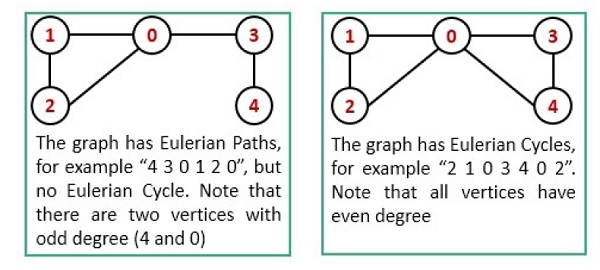# Eulerian Path and Circuit

The Euler path is a path, by which we can visit every edge exactly once. We can use the same vertices for multiple times. The Euler Circuit is a special type of Euler path. When the starting vertex of the Euler path is also connected with the ending vertex of that path, then it is called the Euler Circuit.To detect the path and circuit, we have to follow these conditions −

• The graph must be connected.
• When exactly two vertices have odd degree, it is a Euler Path.
• Now when no vertices of an undirected graph have odd degree, then it is a Euler Circuit.

## Input and Output

Input:
0 1 1 1 0
1 0 1 0 0
1 1 0 0 0
1 0 0 0 1
0 0 0 1 0

Output:
The graph has an Eulerian path.

## Algorithm

traverse(u, visited)

Input: The start node u and the visited node to mark which node is visited.

Output − Traverse all connected vertices.

Begin
mark u as visited
for all vertex v, if it is adjacent with u, do
if v is not visited, then
traverse(v, visited)
done
End

isConnected(graph)

Input − The graph.

Output − True if the graph is connected.

Begin
define visited array
for all vertices u in the graph, do
make all nodes unvisited
traverse(u, visited)
if any unvisited node is still remaining, then
return false
done
return true
End

isEulerian(Graph)

Input − The given Graph.

Output − Returns 0, when not Eulerian, 1 when it has a Euler path, 2 when Euler circuit found

Begin
if isConnected() is false, then
return false
define list of degree for each node
oddDegree := 0

for all vertex i in the graph, do
for all vertex j which are connected with i, do
increase degree
done
if degree of vertex i is odd, then
increase dooDegree
done

if oddDegree > 2, then
return 0
if oddDegree = 0, then
return 2
else
return 1
End

## Example

#include<iostream>
#include<vector>
#define NODE 5
using namespace std;

int graph[NODE][NODE] = {
{0, 1, 1, 1, 0},
{1, 0, 1, 0, 0},
{1, 1, 0, 0, 0},
{1, 0, 0, 0, 1},
{0, 0, 0, 1, 0}
};

/* int graph[NODE][NODE] = {
{0, 1, 1, 1, 1},
{1, 0, 1, 0, 0},
{1, 1, 0, 0, 0},
{1, 0, 0, 0, 1},
{1, 0, 0, 1, 0}
};
*/    //uncomment to check Euler Circuit

/* int graph[NODE][NODE] = {
{0, 1, 1, 1, 0},
{1, 0, 1, 1, 0},
{1, 1, 0, 0, 0},
{1, 1, 0, 0, 1},
{0, 0, 0, 1, 0}
};
*/    //Uncomment to check Non Eulerian Graph

void traverse(int u, bool visited[]) {
visited[u] = true;    //mark v as visited

for(int v = 0; v<NODE; v++) {
if(graph[u][v]) {
if(!visited[v])
traverse(v, visited);
}
}
}

bool isConnected() {
bool *vis = new bool[NODE];
//for all vertex u as start point, check whether all nodes are visible or not
for(int u; u < NODE; u++) {
for(int i = 0; i<NODE; i++)
vis[i] = false;    //initialize as no node is visited

traverse(u, vis);

for(int i = 0; i<NODE; i++) {
if(!vis[i])    //if there is a node, not visited by traversal, graph is not connected
return false;
}
}
return true;
}

int isEulerian() {
if(isConnected() == false)    //when graph is not connected
return 0;
vector<int> degree(NODE, 0);
int oddDegree = 0;

for(int i = 0; i<NODE; i++) {
for(int j = 0; j<NODE; j++) {
if(graph[i][j])
degree[i]++;    //increase degree, when connected edge found
}

if(degree[i] % 2 != 0)    //when degree of vertices are odd
oddDegree++; //count odd degree vertices
}

if(oddDegree > 2)    //when vertices with odd degree greater than 2
return 0;

return (oddDegree)?1:2;    //when oddDegree is 0, it is Euler circuit, and when 2, it is Euler path
}

int main() {
int check;
check = isEulerian();

switch(check) {
case 0: cout << "The graph is not an Eulerian graph.";
break;
case 1: cout << "The graph has an Eulerian path.";
break;
case 2: cout << "The graph has a Eulerian circuit.";
break;
}
}

## Output

The graph has an Eulerian path.